# Introduction

This story presents a special combination of preprocessing and stratification Scikit-Learn`s methods for a classification model training routine.

Data analysis is done over the Kaggle`s classic financial challenge Give Some Credit which requires an algorithm capable of predicting if somebody will experience financial distress in the near future.

A research was conducted over the datasource presenting the attributes available along with their types as well. Data distribution can be previewed as data visualization graphs helping us with decision making as data cleaning routine is performed.

The following routines have as main focus to improve classification metrics results over a specific combination set of Scikit-Learn classification tools, specially selected for this study.

Graphs has been provided along the execution of each step (Figure 1) for better reasoning when making decisions as the study goes on.

By the end of the study we tried to understand how varied methods for data stratification and optimization could be combined together in order to improve a classification model predictions, answering: those methods are capable of returning good results when running over an imbalanced datasource ?.

# Exploratory Analysis

Setting up a modeling and execution strategy is one of the prior tasks that can be performed by a data scientist while exploring and analysing a variety of data characteristics.

Starting with basic questions we seek to answer: (i) how many data points do we have?, (ii) how many data attributes do we have ?, (iv) which types of attributes will we work with ?, (v) is there a value to be predicted ?

Essentials data characteristics are disposed on Table 1 showing us all the eleven attributes that are available for study. Between numerical and categorical attributes we also have the boolean prediction target: SeriousDlqin2yrs.

Seeking for first insights a correlation analysis was performed over those numerical attributes (Figure 1) showing us how they are related among themselves.

Despite the usually correlation interval that relies on -1 and 1 we had an interval from -0.2 to 0.3 to work with. Based on these values and colored legends on Figure 1 it is possible to perform the analysis of features relationships and its intervals.

Positive correlations indicate that when the value of an attribute increases, the value of the related attribute also increases. Negative correlations may indicate that when the value of one attribute increases, the other decreases.

Values that are close to -0.2 or 0.3 were considered relevant and receive special attention during the process. Correlations with values near by 0 can indicate a low relationship between the attributes, values equals to 0 indicates no relationship.

A general analysis over the attributes that are strongly related with the target variable is presented on Table 2 among with additional information about any unexpected correlation between them.

A logic between positive and negative relationships can be observed at this point. Taking the correlation close to -0.2 between Age and NumberOfDependents as an example, meaning that people between 30 and 50 years old tend to be related with a higher the number of dependents, as shown in Figure 2.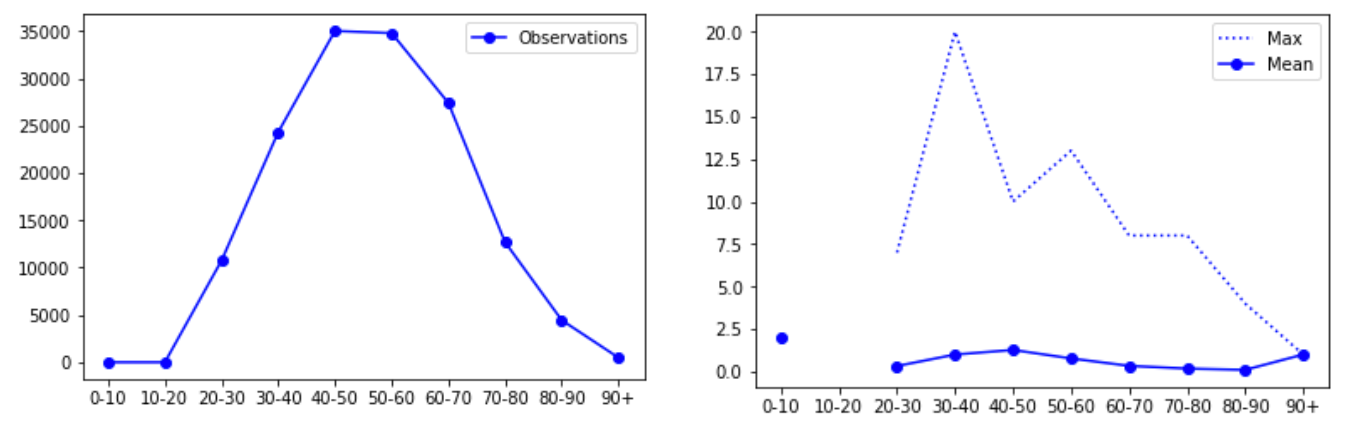Figure 2: Frequency polygon by age values; comparison chart between maximum and average number of dependents by age groups

As said earlier, the SeriousDlqin2yrs attribute will be our prediction target. The values for this attribute are categorical, indicating 0 or 1 for borrowers who have delayed or not 90 days or more in repaying their loans.

Therefore, as explained in the Algorithm Selection section, our case study will be treated as a classification problem. All choices regarding the validation, performance algorithm and metrics refers to this problem category.

Still in the exploratory analysis, we must carry out some relevant tests and observations in the data set. As a first step we check out the distribution corresponding to the both target classes (Yes and No) assuming that we are dealing with a classification problem.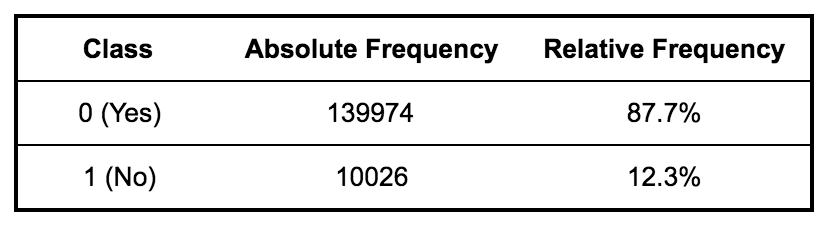Table 3: View of the frequency between the two classesFigure 3: Graphical view of the proportion between specimens of each class

Table 3 and Figure 3 give us the necessary insight to understand that a sample of the data set may have more units of class 0 than of class 1. Such a scenario can result in a model with good accuracy limited to one of the classes, a model that approves loans when it should deny.

A balancing problem occurs when classes are not represented equally. Our data set is not balanced and the ratio between the Class-0 and Class-1 units is approximately 14: 1; 1 unit denied for 14 approved units.

# Data Cleaning

The data cleaning process can be a time consuming task for the data scientist. There is a difficulty in finding content related to the subject, which is an important step in a machine learning project.

This stage precedes the training stage. At that moment, the data scientist is concerned with reproducing the best version of the data set under study. Thus, it is expected that quality data, once submitted in the training stage, will produce quality results.

There is no data cleaning guide that covers any study of a data set. The strategies will vary from one set to another. However, some basic precautions can be taken, regardless of the problem under study.

The first step we took was to treat missing values for the Age and MonthlyIncome attributes. We add an observation flag indicating when the value has been omitted and then fill in the original value with zero.

This technique, known as flagging and filling, allows the algorithm to estimate the best constant for cases of data omission. We did not choose to fill the missing values with the average value in order to avoid possible loss of information.

Moving on to the relationship observed between the Age and NumberOfDependents attributes, we can see that although there is a pattern of decrease in dependents when advancing the borrower’s age, in addition to the outliers for the NumberOfDependents attribute.

Therefore, it is necessary to evaluate the frequency of these observations in relation to the total data of our case study. For this, we search for all borrowers with more than 5 dependents and calculate the frequency of each group, as shown in Table 4.

It is possible to verify that the values are very low when compared with the total number of borrowers. For this same reason, the table is the best tool to provide this information, when compared to a histogram, since small values are imperceptible in the large dimension of the data volume.

Therefore, we chose to dispense with records that represent less than 0.1% of the volume of data, in order to avoid possible side effects in models sensitive to outliers. Borrowers with more than 6 dependents were excluded from the training set.

Following the same principle, we decided to study the distribution of borrowers by age ranges. Table 5 shows that there are records below the age of 20 and above 99, which represent 0.005% of the total volume of data. Therefore, borrowers in these two age ranges were also excluded from the training set.

# Feature Engineering

Before training our model, we chose to perform a minimal attribute engineering task. Like data cleaning, this phase can positively impact the performance and accuracy of the model studied.

There are infinite options for manipulating attributes because such a process requires a lot of business knowledge, making a process of setting standards more complicated, requiring more creativity and problem mastery.

We can resort to some common practices in this part of the process, one of which is the creation of interaction attributes. In our context, interaction attributes represent product, sum or difference between two attributes.

Figure 1 is a visual notation for the relationship between the attributes of our case study. We chose to add the pairs of attributes that have a positive relationship with each other, keeping the values in an interval between 0 and 1.

We chose to produce the PastDueScore attribute, which is the sum of the NumberOfTime30–59DaysPastDueNotWorse and NumberOfTime60–89DaysPastDueNotWorse attributes. As shown in Table 1, the two attributes are indicators of late payment. The sum of the two allows us to observe both delay indicators at the same time.Formula 1: Normalization of data in a range between 0 and 1

In order for the values of the PastDueScore attribute to represent a degree of debt in a given range, we decided to normalize the sum result, applying Formula 1. Thus, the degree of debt is measured in an interval between 0 and 1, where 0 is less degree of debt and 1 the highest degree.

# Training

The classification model training process will be performed on a training sample extracted from the original data set; a smaller portion of the set will also be separated for quality testing.

The test and training samples are also useful to assist in parameter adjustments and the use of different methods during the training cycle, also supporting the production of performance graphs. The cut-off values experienced for the test sample were 0.22 and 0.33.

Although a set of tests is available through Kaggle, it has the purpose of producing the entry for the evaluation of the trained model’s public score. Unlike the test sample that we are producing, the previous one cannot be used to extract metrics.

With the purpose of stipulating a basic performance metric, we started the training routine with a naive classification model, without specific adjustments of performance parameters but with the adjustments of data already made. The quality of the results is shown in Figure 4.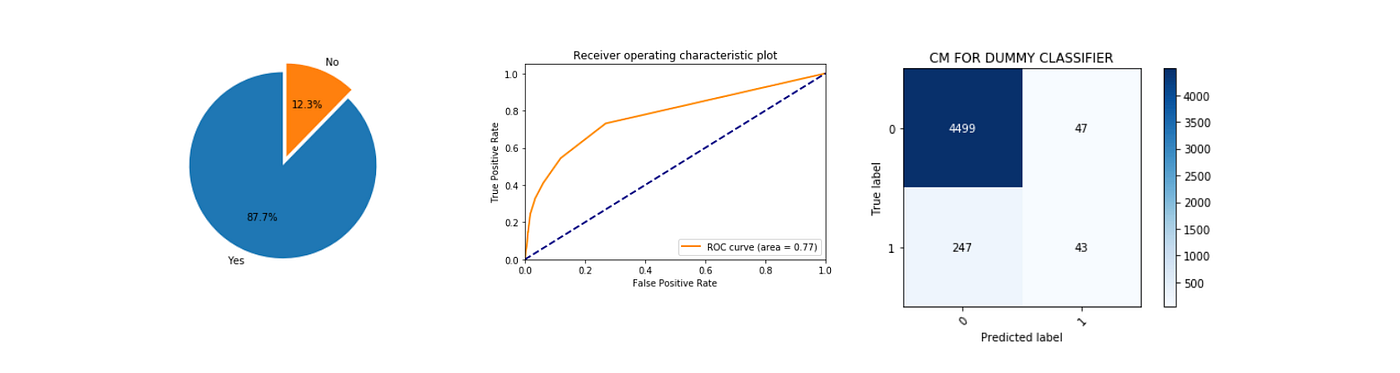Figure 4: Performance results of the naive classifier for class-balanced stratification method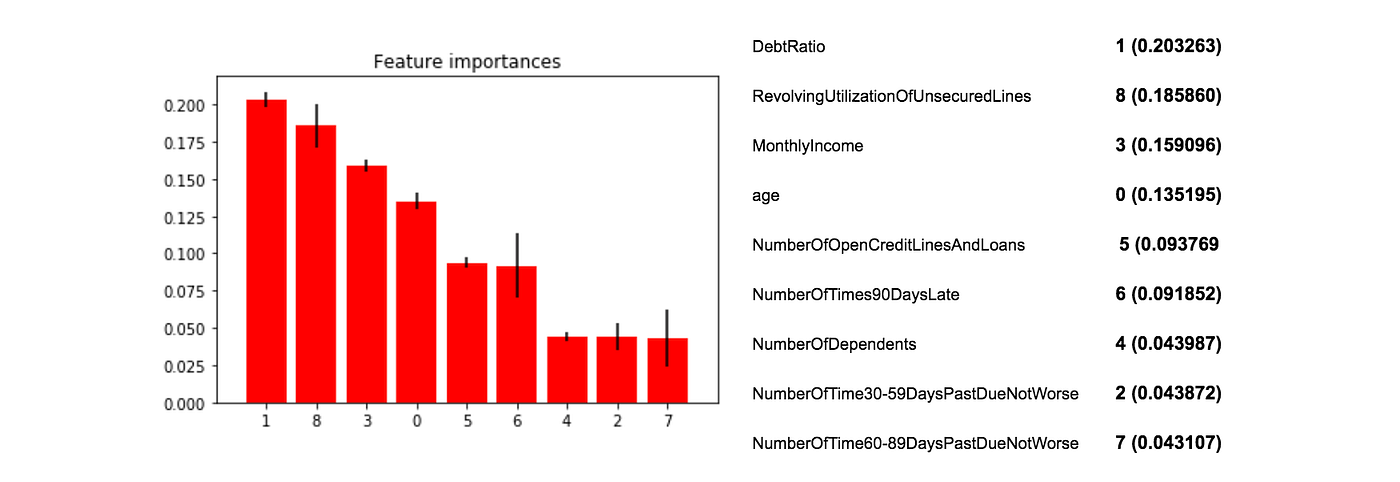Figure 5: View of the importance of the original attributes of the data set

Figure 4 shows the proportion between classes in the training set, the ROC curve as a classification metric and the confusion matrix, given the quality of the naive model’s output, in the main diagonal we have the values for the correct predictions of each class, in counter-diagonal we have the values wrongly classified.

Once with the values of the dummy model in hand, we have the first reference values for optimization in the training process. We will start working on the AUC metric, looking for better values than 0.77.

In Figure 5 it is possible to see the importance of each attribute in the classification process of our dummy model. Attributes with values greater than 0.04 will be selected for the next training cycle.

After conducting the expertise on the original attributes of the data set, we performed the same importance analysis process, Figure 6, attaching the attributes imputed in the attribute engineering cycle and excluding those of lesser value observed in Figure 5.

Using the set of tests provided by Kaggle, we trained the naive model with the new selection of attributes and submitted the results of submissions to Kaggle. Table 7 shows the results obtained by the naive model, in ascending order of score.

Kaggle offers access to the Leaderboard with scores values in descending order. We will use these values as a benchmark for the performance of our classification model. The score of 0.767 places our model among the eight hundred positions in a total of nine hundred.

Among the eight hundred positions, there are those that reached a score of at least 0.80. Thus, we assume that our model could still achieve better results since we can still perform improvement tasks, such as adjusting hyperparameters.

Therefore, at this point, we can define the first goals for improving the model: (i) reduce the values of the diagonal of the confusion matrix; (ii) seek the basic cut line of the public Kaggle score of at least 0.80 with the hyperparameter adjustments.

A model with the correct configuration of hyperparameters can have its performance optimized. The formulation of hyperparameters must occur before the training stage of the model, as such information is not extracted from the data set, but rather determined by the data scientist.

The hyperparameter adjustments occur over the domain of the chosen model, in our case, the Random Forest. The first optimization cycle takes place on the total number of decision trees and the total attributes available for each tree when executing the branches.

We used a randomized search method called RandomizedSearchCV, from the Scikit Learn library, for the optimal choice of the set of hyperparameters in our case study. Table 7 presents the optimal choice obtained after the execution of the method, in the entire training set.

We tested the model selected using the RandomizedSearchCV method so that the results were compared with those obtained in the first training cycle. As expected, our model achieved an improvement in its performance, reaching the mark of 0.82 in the public Kaggle score.

The mark achieved is the best expected minimum of our application after the steps followed so far. At this point, we raised the question: is it possible to go beyond the result presented and match the best placed ?. For that, it is necessary to improve our score by 0.0468.

Figure 7 illustrates the confusion matrix given the output of the optimized model. At this point, reinforcing our intention to seek the best parameters for our model, it becomes necessary to implement performance monitoring routines for our model during the training phase, ensuring that we are on a correct path.

As mentioned earlier, for testing purposes we have separated out a portion of the original training set provided by Kaggle. With that, it is possible to extract the probabilistic estimates of the positive classes and compare with the real expected frequency of that same positive class.

Figure 8 illustrates the ability to classify the new model. We obtained 0.83 as the area value below the ROC curve and compared it with the result processed by Kaggle himself, resulting in approximately the same value 0.83 according to Table 8. The Model Evaluation session gathers more information about the AUC metric.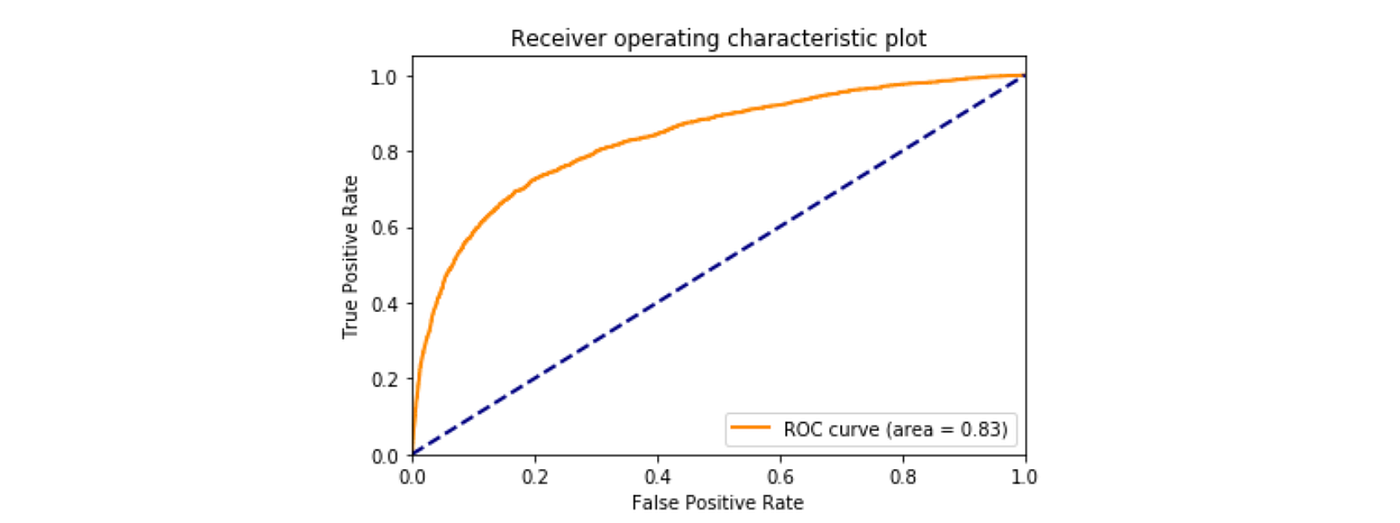Figure 8: Performance quality of a classification model using the AUC method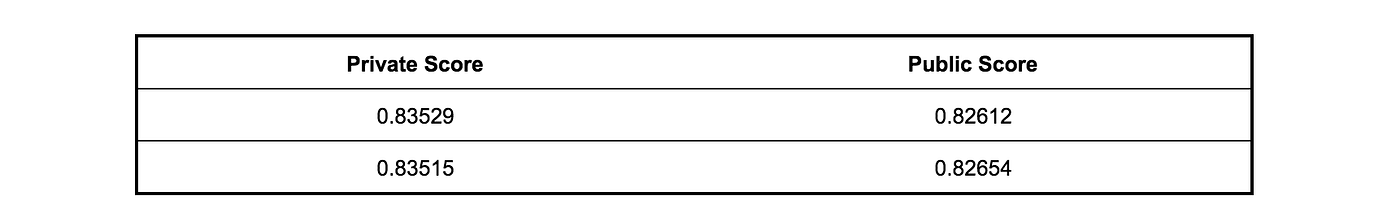Table 8: Evolution of the score computed by Kaggle after the new training routine

We still have a few options to explore in order to optimize our model’s predictive quality. We can test new parameters in the optimization routine or return to the Attribute Engineering phase, in order to improve the quality of data entry.

We first chose to proceed with the testing of new parameters in the optimization routine. Table 9 and Figures 9 and 10 show the metrics obtained in each test cycle. First, we added the criterion attribute as a grid option, so that the gini or entropy options could be tested.

At the same time, we alternate the metrics of the optimizer, between Recall and Precision, assuming that our distribution between classes is not balanced, for comparison purposes. The results are shown in Table 9, where it is possible to notice a small difference in quality between the two outputs.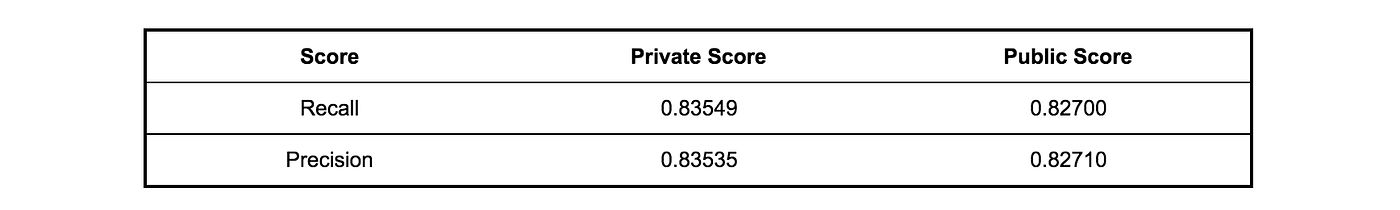Table 9: Result obtained after changes in the parameter optimization methods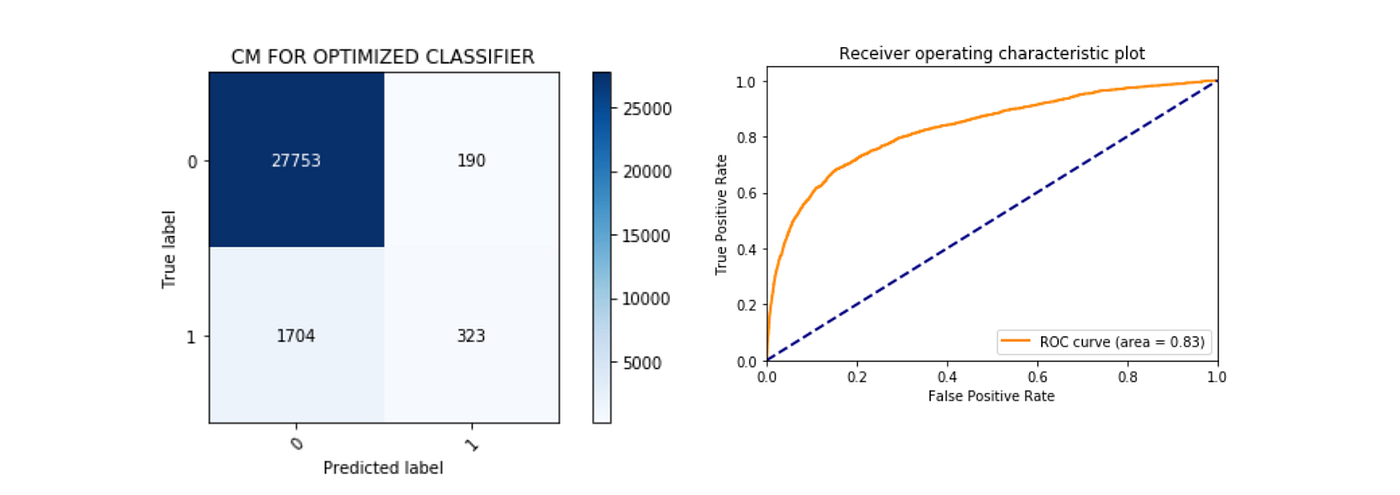Figure 9: Confusion matrix and ROC AUC curve for the second optimization phase. Scoring Recall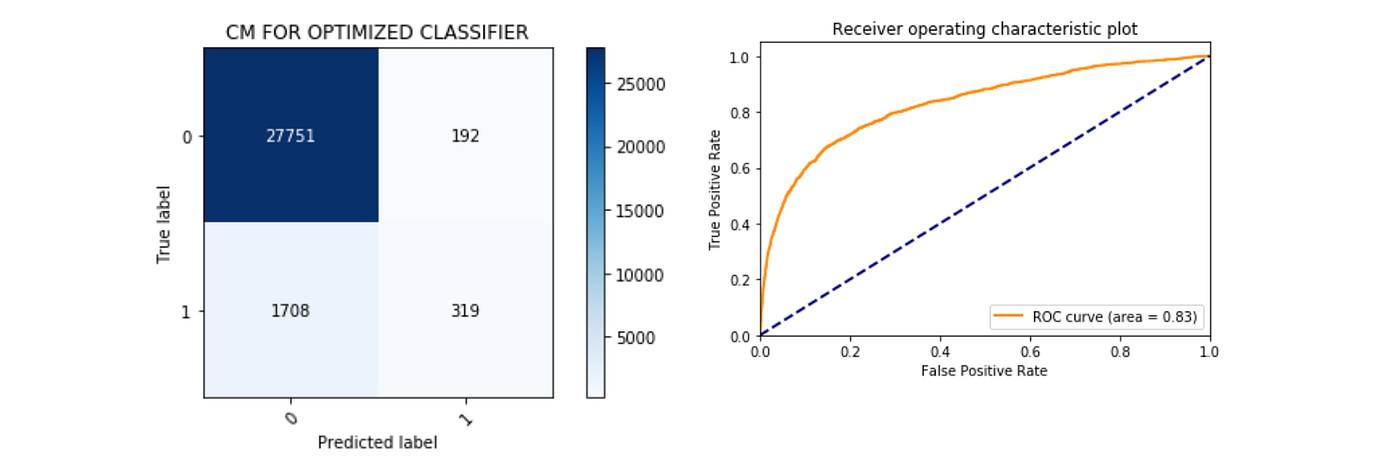Figure 10: Confusion matrix and ROC AUC curve for the third optimization phase. Scoring Precision

One possible way would be to concentrate efforts on the accuracy of the minority class; for this, the scoring parameter will be fixed in precision and we repeat the process of selecting the best parameters by configuring a set of values for the class_weight attribute of our classification model.

The class_weight parameter of the Random Forest algorithm allows associating weights to the data set classes; when not informed, the algorithm assumes that each class has a weight of 1, that is, equal. Table 10 shows the optimal values of hyperparameters after the inclusion of the class_weight attribute.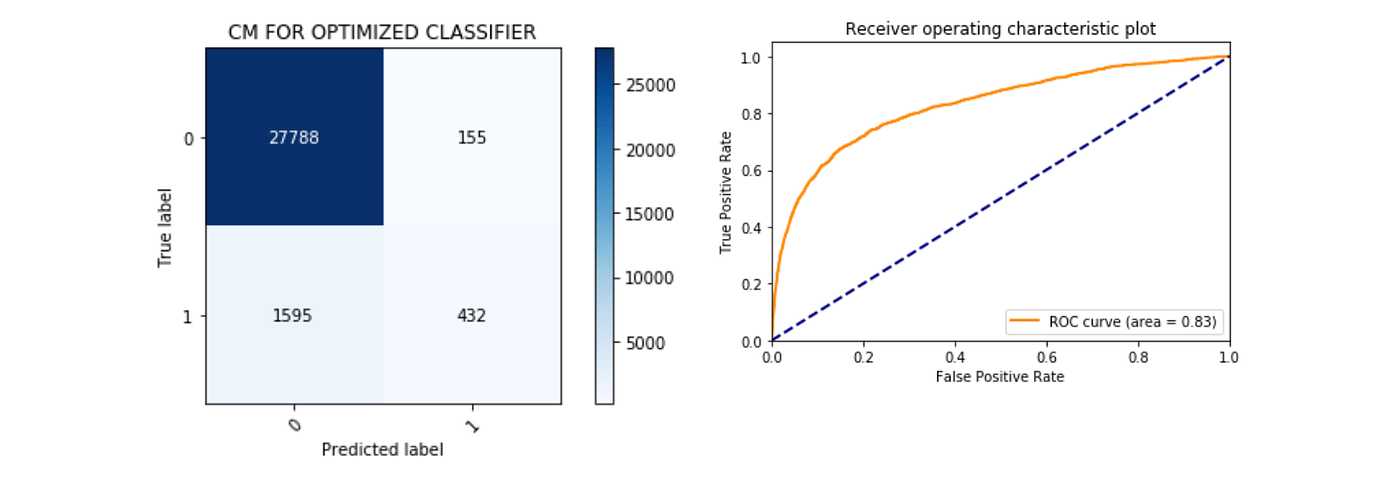Figure 11: Confusion matrix and ROC AUC curve for optimization with class imbalance parameter. Scoring Precision

As shown in Figure 11, we obtained the expected reduction in the values of the diagonal of the Confusion Matrix. However, it is possible to observe that such changes did not impact the quality of classification of the model in the perspective of the ROC curve.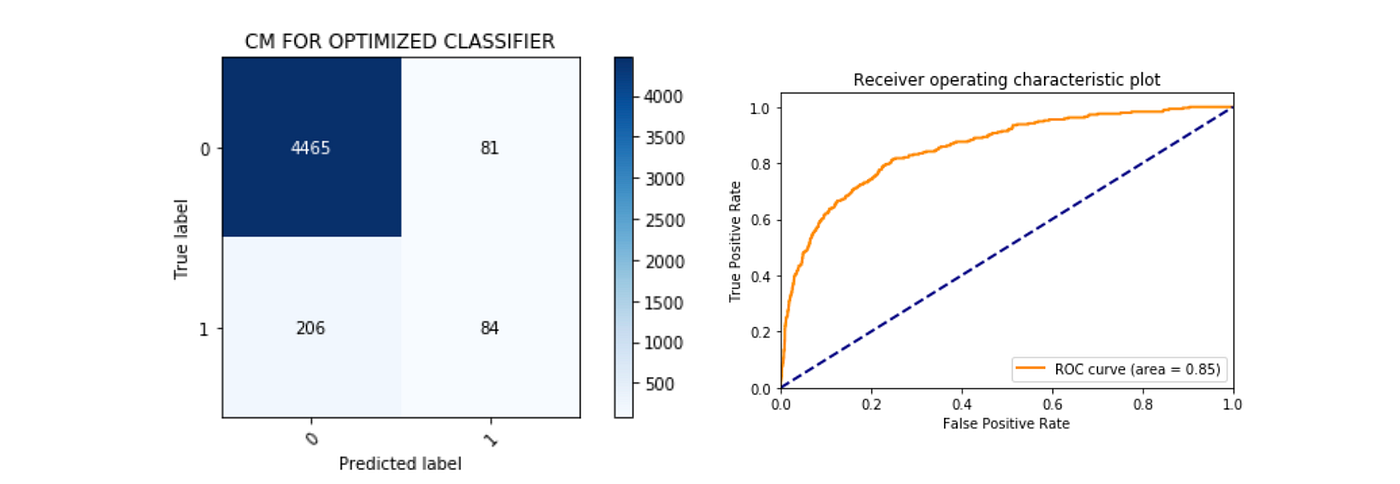Figure 12: Confusion matrix and ROC AUC curve for optimization with different score parameters and weights between classes

We conclude that alternating the Accuracy and Precision metrics in the optimization cycle does not reflect significant changes in the Kaggle score evaluation. We propose to exhaust the methods of attempting optimization with a new set of parameters according to Table 11.

The differences from this last cycle to the previous ones occur in the fact that we indicate a list of scores to be evaluated during the optimization of hyperparameters, as well as the indication of a refining metric (refit).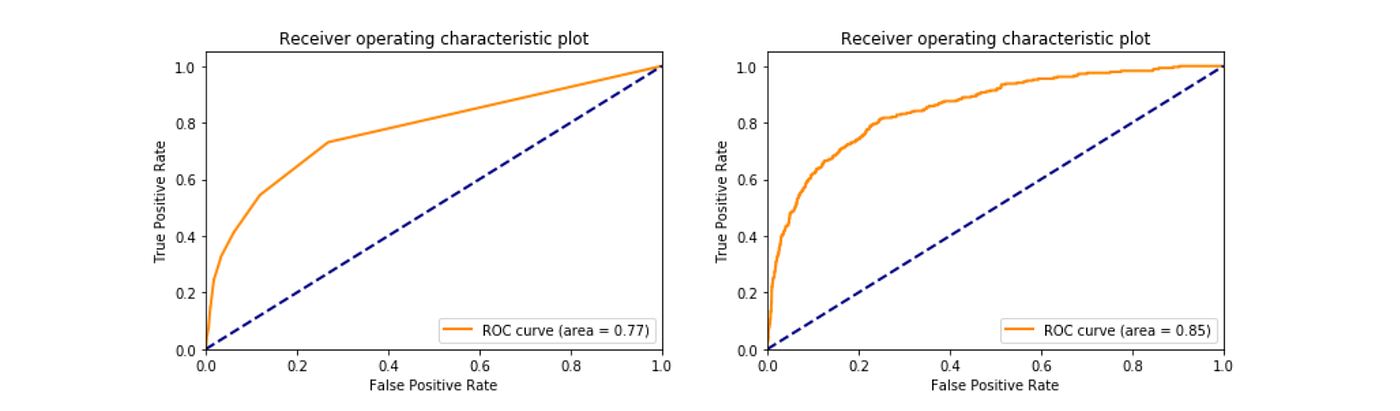Figure 13: Performance comparison between the naive model and the optimized model using the ROC curve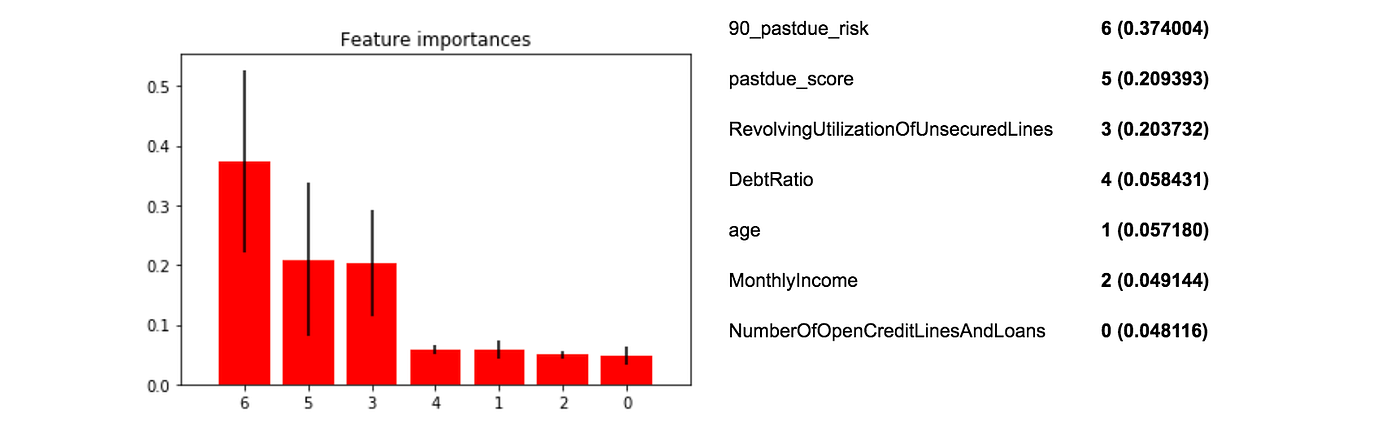Figure 14: View of importance of attributes of the optimized model

Figure 13 compares the performance of the naive classifier with that of the optimized classifier. Areas above 0.8 represent fairly good discriminatory ability [•]

The quality improvement obtained through laborious selection of attributes during optimization was huge, as well as the importance of maintaining attributes during training cycles.